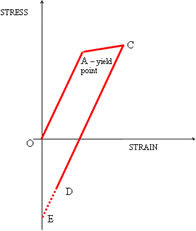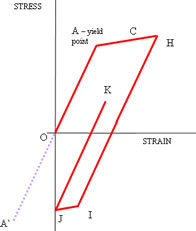## Nominal and Non-linear Stresses-Part 2# Nominal and Non-linear Stresses - Part 2

## Cyclic Plasticity

This article extends the introduction to modelling plastic or post-yield material behaviour introduced in the previous article. Plastic stresses first occur at stress concentrations or notches. These are termed secondary stresses in the context of the pressure vessel codes. Important concepts in cyclic or fatigue loading of components where the yield stress is exceeded will be explained.High cycle fatigue refers to problems when the number of cycles to failure (or life) typically exceeds 105. In order to sustain this number of stress cycles, peak stresses are substantially lower than the yield strength of the material. This is therefore a simpler problem in principle than dealing with plastic cyclic stresses. The well known stress-life (or S-N) curve is pivotal in determining high cycle fatigue lives. In Figure 1, repeated from the previous article, this corresponds to stress cycles along the line OA. When stresses are high enough to cause plasticity to change during the load history, no equivalent of the S-N curve is available. Referring to Figure 1 again, if the yield point is exceeded, the stresses on unloading will follow a line parallel to OA. The diagram shows a particular case of loading to point C and the corresponding unloading to a negative residual stress and positive residual strain, at D.

At points within and around a substantial yielded region, the unloading (or the position of D) is not usually returned to either a zero stress or zero strain state, due to the constraint effects of the surrounding material. However, if the region of yield is small, which is consistent with the concept of secondary stress, the surrounding structure forces the region to a state of zero strain, indicated by point E in the diagram.

Considering the case of full unloading to zero strain at E, subsequent repeated application of the load will cause the straight line stress strain curve EC to be followed. So, after an initial over stress at this point in the component, subsequent cyclic stress cycles are elastic and the fatigue life should be close to that predicted by the high cycle S-N fatigue curve. However, at some peak stress higher than C, plasticity will occur during both unloading and subsequent reloading. In pressure vessel applications, it is often required to determine whether such plastic cycling is stable. Repeated applications of a cyclic load will result either in the plastic region expanding through the component section on each load cycle until failure occurs (perhaps after a very few cycles), or until the size of the plastic region stabilises with a higher or much higher number of cycles to failure. These are respectively termed ratchetting and shakedown.In Figure 2, the line HIJ represents the stress-strain excursion from peak load to zero strain. This new shaped unloading line is due to the peak stress H, which is greater than C in Figure 1. The first portion of the unloading stress-strain curve HI is, like curve CE, parallel to the elastic line OA. Line HI is the same length as A` A, where A` represents the negative yield point. As the small region of plasticity is driven to zero strain by the surrounding material, what might be called the unloading elastic limit is reached at point I. The remainder of the trajectory to zero strain is therefore reached along the plastic line IJ, which is parallel to AH.

Subsequent reapplication of loading will follow the stress-strain curve towards K. The important difference between the two diagrams is that reloading in Figure 2 defines a new stress-strain path JK rather than following the previous path. This effect is called plastic cycling or hysteresis – the important phenomenon here is that plastic work is occurring to the material not only for the first cycle but also in subsequent cycles. Detailed numerical analysis is the only option to establish whether the plastic cycling is stable or propagates throughout the section, leading to failure or gross deformation.

The geometry of these simple stress-strain curves can be investigated to show that the limiting peak strain at which the transition to plastic cycling occurs (the transition between the first and second diagrams) is twice the yield strain. This is a useful conservative working figure used in the pressure vessel codes for guarding against ratchetting.